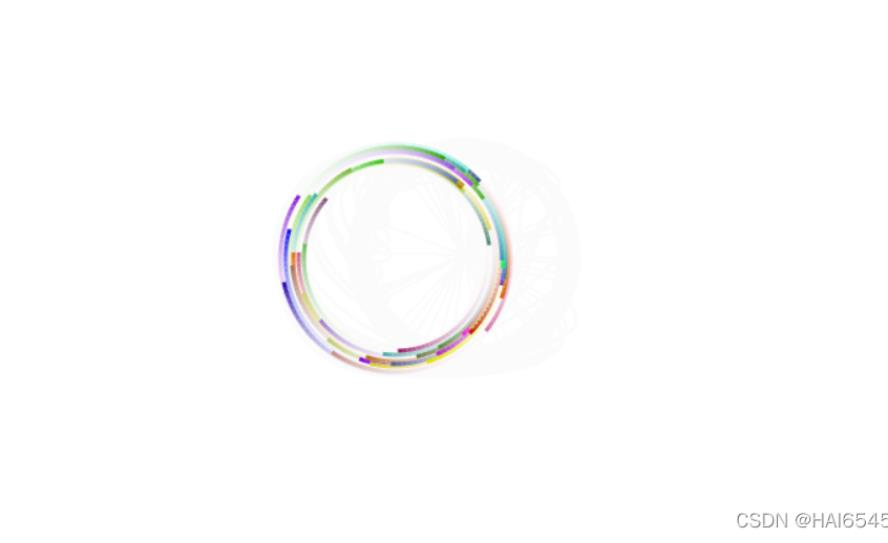# JavaScript实现环绕鼠标旋转效果

```<!DOCTYPE html>
<html lang="en">

<meta charset="UTF-8">
<meta name="viewport" content="width=device-width, initial-scale=1.0">
<meta http-equiv="X-UA-Compatible" content="ie=edge">
<title>Canvas Resize</title>
<style type="text/css">
/* 清除默认边距 */
*{
margin: 0;
}
html,body{
/* 设置浏览器宽高为100% */
width: 100%;
height: 100%;
}
</style>

<body>
<!-- canvas可以书写内容 但是只会在浏览器不显示canvas时才会显示书写的内容 -->
<canvas></canvas>
<script src="js/javascript.js"></script>
</body>

</html>```

js：

```//以前封装的函数 直接从工具库中复制过来的
/**
* 获取随机数的函数 获取到的随机数取整
*/
function getRandom(a, b){
return Math.floor(Math.random() * Math.abs(a - b)) + Math.min(a, b)
}
/**
* 获取16进制的随机颜色值
*/
function getColor(){
var color = '#'
for(var i=0;i<3;i++){
var hex = getRandom(0, 256).toString(16)
color += hex.length === 1 ? '0' + hex : hex;
}
return color
}
//获取随机数 但是不取整
function randomDoubleFromRange(a,b) {
return Math.random() * (Math.abs(a - b)) + Math.min(a, b);
}

//设置鼠标的位置 先设置为window的中心
var mouse = {
x: window.innerWidth / 2,
y: window.innerHeight / 2
}

//创建鼠标移动的事件监听事件  监听鼠标所在window中的实时位置
//给数组中的x和y修改值
mouse.x = window.clientX;
mouse.y = window.clientY;
});

//当窗口大小发生改变时 重新获取画布宽高并且重置代码 resize事件在窗口大小变化时触发
//window的可用窗口大小 包含了滚动条的区域大小
canvas.width = window.innerWidth;
canvas.height = window.innerHeight;
init();
});

//获取页面上的canvas
var canvas = document.querySelector('canvas');

//设置canvas的大小为window大小 如果不设置 就使用默认宽高300*150
canvas.width = window.innerWidth;
canvas.height = window.innerHeight;

//设置canvas绘画的环境 语法Canvas.getContext(contextID) 现在版本只能写参数2d 以后可能会支持3d
var ctx = canvas.getContext('2d');

//封装一个制造环绕鼠标的小球的函数 参数是小球圆心在x轴的位置 在y轴的位置 小球半径 小球填充颜色
function Particle(x, y, radius, color) {
//小球中心点的x轴位置
this.x = x;
//小球中心点的y轴位置
this.y = y;
//小球半径
//小球颜色
this.color = color;
//小球转动的弧度值 不取整 如果取整 就少了很多数字 很多小球都会重叠
this.theta = randomDoubleFromRange(0, 2 * Math.PI);
//小球绕中心点转动的速度
this.speed = 0.05;
//小球距离中心点的距离
this.distance = getRandom(70, 90);
//小球跟随鼠标移动的速度
this.dragSpeed = 0.05;
//记录鼠标移动前鼠标的位置
this.lastMouse = {
x: x,
y: y
};
//绘制函数
this.draw = function (lastPosition) {
//重置当前路径 因为创建的每一个路径都会以上一个beginPath之后的所有路径作为基础绘制 会把之前所有线条的颜色全部绘制成和最后一个线条相同的一个颜色
ctx.beginPath();
//将小球颜色作为填充颜色
ctx.strokeStyle = this.color;
//将小球半径作为路径宽度
//路径起始位置
ctx.moveTo(lastPosition.x, lastPosition.y);
//路径结束位置
ctx.lineTo(this.x, this.y);
//绘制确切路径
ctx.stroke();
//关闭路径 不是结束路径 而是从结束点创建一条线连接到起始点 使路径闭合
ctx.closePath();
}

//更新数据的函数
this.update = function () {
//创建lastPosition对象接收上一次鼠标的x和y的值
var lastPosition = {
x: this.x,
y: this.y
}

//每次调用函数移动鼠标当前位置和上一次位置之间的dragSpeed = 0.05的距离 产生拖拽感觉
this.lastMouse.x += (mouse.x - this.lastMouse.x) * this.dragSpeed;
this.lastMouse.y += (mouse.y - this.lastMouse.y) * this.dragSpeed;

//更新小球当前位置 因为每一次调用小球的旋转角度不同导致其位置不同
this.x = this.lastMouse.x + Math.cos(this.theta) * this.distance;
this.y = this.lastMouse.y + Math.sin(this.theta) * this.distance;

//更新小球的角度值
this.theta += this.speed;
//将参数传递给绘制函数绘制路径
this.draw(lastPosition);
}
}

//定义particles变量
var particles;

//初始化函数
function init() {
// 每次调用这个函数都要先把数组内容清空 因为使用这个函数除了打开网页后第一次代表调用之外 别的调用都表示窗口大小发生了改变 参数发生了变化 为了修改窗口大小之后又因为调用该函数导致小球重复创建 所以要调用后先清空再创建
particles = [];
// 绘制50个小球
for (var i = 0; i < 50; i++) {
//获取随机的颜色
let color = getColor()
//将构造函数创造的小球添加到数组中
particles.push(new Particle(canvas.width / 2, canvas.height / 2, 3, color));
}
}

function animate() {
//定时的调用这个函数 类似于setInterval 但是setInterval时间间隔不是很准确 requestAnimationFrame固定为以每秒60次的频率调用一次括号内的函数
requestAnimationFrame(animate);

// 每一帧都给之前的帧蒙上一层白色透明的矩形 用以清除上一帧绘制的路径
//填充矩形颜色 矩形背景颜色 透明度设置为0.1 可以使之前几帧的路径因为多次覆盖慢慢边淡 只是会在背景上留下很淡的痕迹 如果直接使用rgb白色覆盖 虽然没有痕迹残留 但是之前路径直接被白色覆盖 没有拖尾效果
// ctx.fillStyle = 'rgb(255, 255, 255)';
ctx.fillStyle = 'rgba(255, 255, 255, 0.1)';
//绘制矩形 给window绘制一个等高等宽的矩形 以此制造一个渐变的效果
ctx.fillRect(0, 0, canvas.width, canvas.height);

//对键名遍历数组 获取canvas.width / 2, canvas.height / 2, 3, color参数 每遍历一次就调用一次update函数 将获取到的参数作为实参传递给update函数
for (var p of particles) {
p.update();
}
}

//初始化 创建小球
init();
//开始动画
animate();```• js实现图片旋转 js滚动鼠标中间对图片放大缩小
• touch.js 拖动、缩放、旋转 （鼠标手势）功能代码
• 基于css3新属性transform及原生js实现鼠标拖动3d立方体旋转
• 图片旋转、鼠标滚轮缩放、镜像、切换图片js代码
• js根据鼠标移动速度背景图片自动旋转的方法